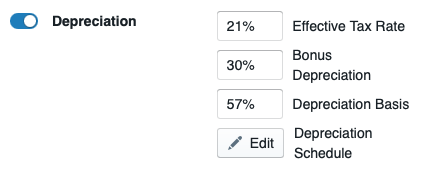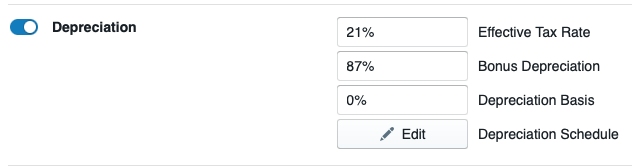# How to Model Depreciation

While investing in solar, businesses look for ways to reduce their payback and boost their financial metrics. Depreciation facilitates this by allowing solar investors to "write down" the cost of a tangible asset (such as equipment) over its useful life span for accounting and tax purposes.

Once a Financial Model has been created with Depreciation as an acceptable input, you can follow this video to see how to model Depreciation in HelioScope.

After Selecting Depreciation in your Financial Model, If modeling the 30% Bonus Depreciation you will have to input the following fields:If modeling the 100% Bonus Depreciation please see the example in the image belowEffective Tax Rate: The combined tax rate that drives the tax benefit for the depreciation calculation. It can be the Federal Tax Rate, the State Tax Rate, or a combination of the two.Bonus Depreciation: Bonus depreciation occurs in year 1. Note: this is operating in absolute percentage. If you want 87% of the system to be depreciated and 30% of the system price to be depreciated in year 1 as bonus depreciation, you will set Depreciation Basis = 57% and Bonus Depreciation = 30%

Depreciation Basis: Amount of the total system price that is eligible for a depreciation schedule. Currently in the US, set at 87%.

Depreciation Schedule: Defines the schedule upon which the project is depreciated. The user sets up the number of years and the percentage of depreciation in each year. Note: regardless of Depreciation Basis %, the annual schedule should add up to 100% because each year is a percentage of the basis.

You can also create your own  Financial Model and include Depreciation as part of it.

Note: Countries model Depreciation according to their own rules and legislation. We suggest developers verify local guidelines before modeling them in HelioScope.